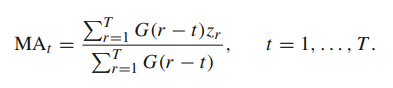## SAS IML: how to create a T x T matrix from a T x 1 vector

Hi everyone,

I have a column vector (1 2 3 ... 20 000). I would like to know how to create  a 20 000 x 20 000 matrix which columns correspond to the first column vector I mentioned.

Thank you 🙂

1 ACCEPTED SOLUTION

Accepted Solutions

## Re: SAS IML: how to create a T x T matrix from a T x 1 vector

I think you can use the IML REPEAT statement.

If you have named the column vector you already have to be X, then you want

``want_matrix = repeat(x,1,20000);``
--
Paige Miller
7 REPLIES 7

## Re: SAS IML: how to create a T x T matrix from a T x 1 vector

I think you can use the IML REPEAT statement.

If you have named the column vector you already have to be X, then you want

``want_matrix = repeat(x,1,20000);``
--
Paige Miller

## Re: SAS IML: how to create a T x T matrix from a T x 1 vector

@PaigeMiller thank you for your answer ! I tried and the following message appeared: "unable to allocate sufficient memory" ... 😅

## Re: SAS IML: how to create a T x T matrix from a T x 1 vector

You will need about 3GB to store a 20000x20000 matrix and this is probably more than your default memory allocation for SAS.  To see run:

``````proc options option=memsize value;
run;``````

There is a command line option -MEMSIZE that you use when starting the SAS application to increase the allocation.  Alternatively, describe why you need to create such a large matrix, and what you want to do with it, then perhaps someone can suggest a way of doing it that needs less memory.

## Re: SAS IML: how to create a T x T matrix from a T x 1 vector

I am in complete agreement with @IanWakeling .

@_vichz Please explain to us why you need such a huge repetitive matrix. What are you going to do with it?

--
Paige Miller

## Re: SAS IML: how to create a T x T matrix from a T x 1 vector

First of all, thank you for taking the time to answer my question 🙂I have to compute the moving average of a financial time series in order to detrend it. Here, G is the density of a gaussian (mean=0, standard deviation=10). I thought I could do that using matrices. Initially, I took the whole time series (approximately 20 000 observations). Now I sampled it and took the "interesting" part (500 observations).

## Re: SAS IML: how to create a T x T matrix from a T x 1 vector

If you have a license to SAS/ETS, moving averages can be computed by PROC EXPAND.

https://documentation.sas.com/doc/en/pgmsascdc/9.4_3.4/etsug/etsug_expand_details19.htm#etsug_expand...

--
Paige Miller

## Re: SAS IML: how to create a T x T matrix from a T x 1 vector

It is unlikely that the value at time t=20000 times series is related to the value at t=1. Typically, correlations are observed for small time values such as 1-7 (daily), 30 (monthly), or maybe 365 (annually). It might be that you are sampling too frequently (eg, microseconds) and you should decrease the sampling rate.

Most time series procedures in SAS create the lagged effects internally, so there is rarely a need to create a matrix whose columns are the lagged effects. However, to answer your question, you can use the LAG function in SAS IML to create a matrix that contains the lagged effects:

``````proc iml;
v = T( 1:10 );       /* input vector */
Lags = lag(v, 0:5);  /* matrix of lags 0,1,2,... */
print Lags;
``````

From The DO Loop